maths > vector-algebra

Parallelogram Law

what you'll learn...

Overview

»  continuous addition of $\stackrel{\to }{x}$$\vec{x}$ and $\stackrel{\to }{y}$$\vec{y}$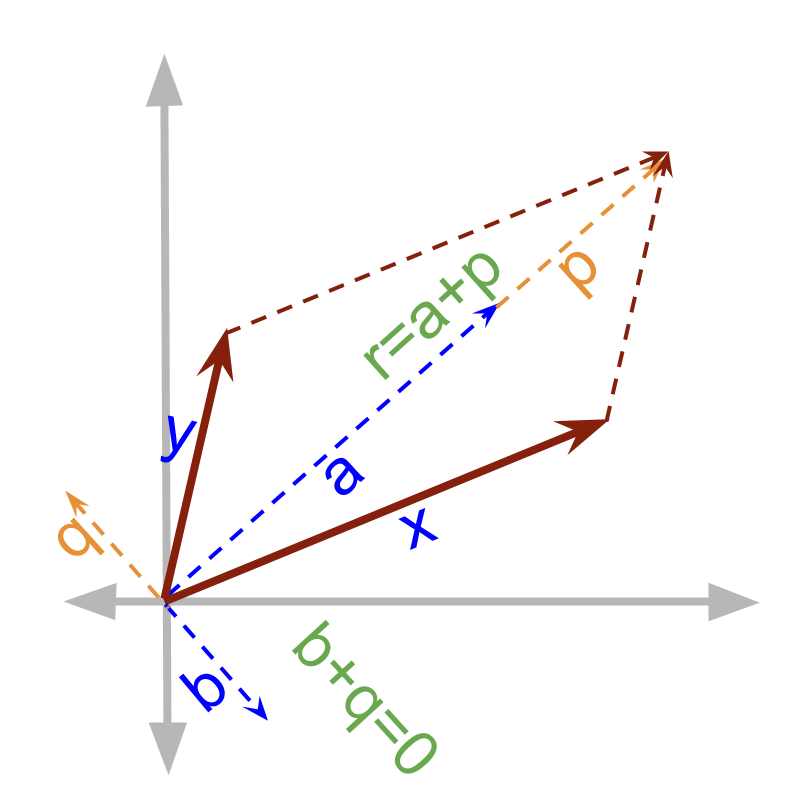→  $\stackrel{\to }{x}=\stackrel{\to }{a}+\stackrel{\to }{b}$$\vec{x} = \vec{a} + \vec{b}$

→  $\stackrel{\to }{y}=\stackrel{\to }{p}+\stackrel{\to }{q}$$\vec{y} = \vec{p} + \vec{q}$

→  the co-initial diagonal of the parallelogram formed by sides $\stackrel{\to }{x}$$\vec{x}$ and $\stackrel{\to }{y}$$\vec{y}$

Note: Using Geometry, the triangle property of vector addition can be extended to explain parallelogram property of vector addition. In the coming pages, we establish a different point of view for the same.

Two taps simultaneously fill a bucket. One fills at $20$$20$ liter per hour and another fills at $10$$10$ liter per hour. This addition is called continuous addition as the quantities are added continuously over time. Scalar quantities can be added continuously..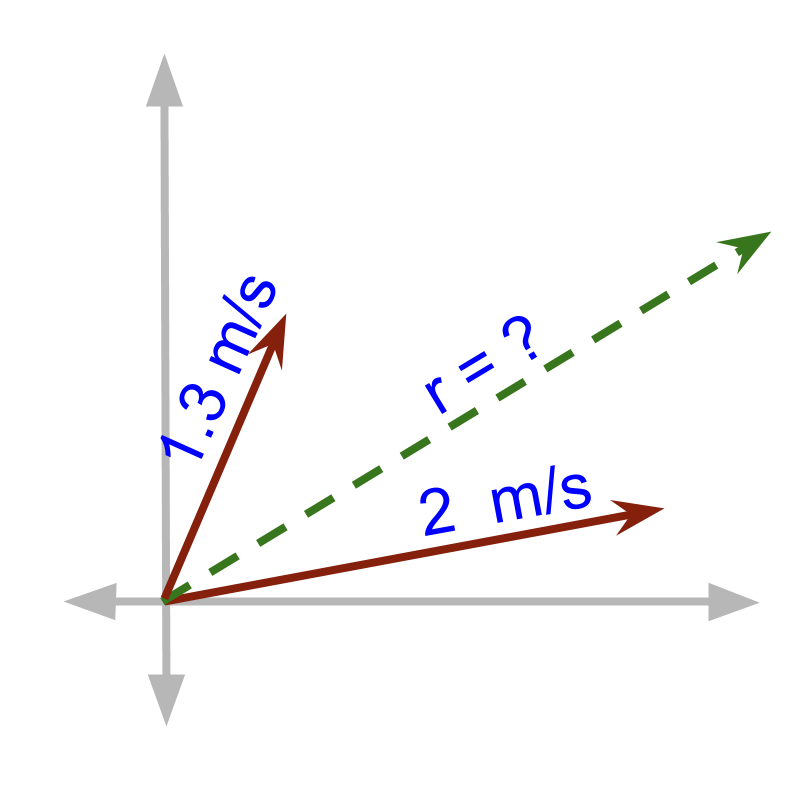A ball rolls with two velocity components $2$$2$m/sec at ${11}^{\circ }$${11}^{\circ}$angle and $1.3$$1.3$m/sec at ${67}^{\circ }$${67}^{\circ}$ angle. The effective velocity of the ball is calculated using Continuous addition of vectors. The two vectors are acting together resulting in a vector sum.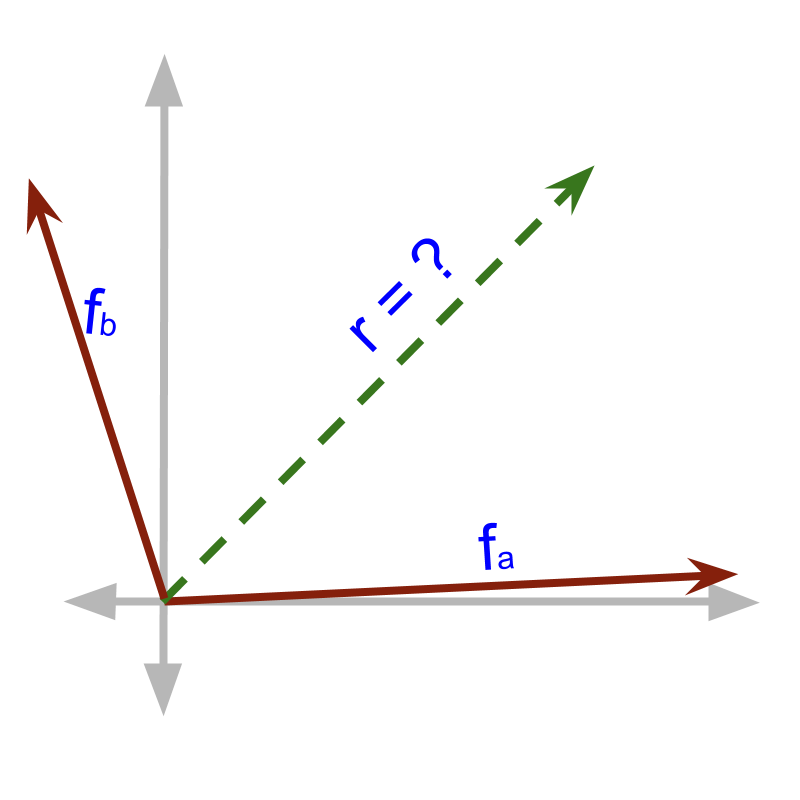A table is pulled by two persons with forces ${f}_{a}$${f}_{a}$ and ${f}_{b}$${f}_{b}$ with an angle $\theta$$\theta$ between the two forces. The effective force on the table is calculated using Continuous addition of vectors. The two vectors are acting together resulting in a vector sum.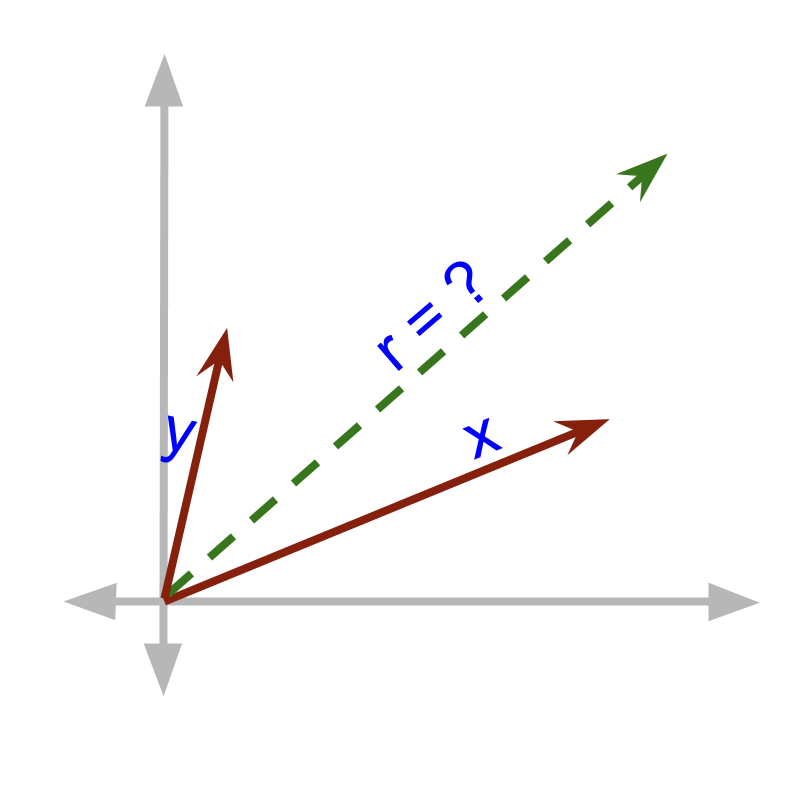Continuous addition of two vectors is shown in the figure.
The magnitude of a vector is equivalently shown as length of the ray in a coordinate plane. Let us see how to calculate $\stackrel{\to }{r}$$\vec{r}$.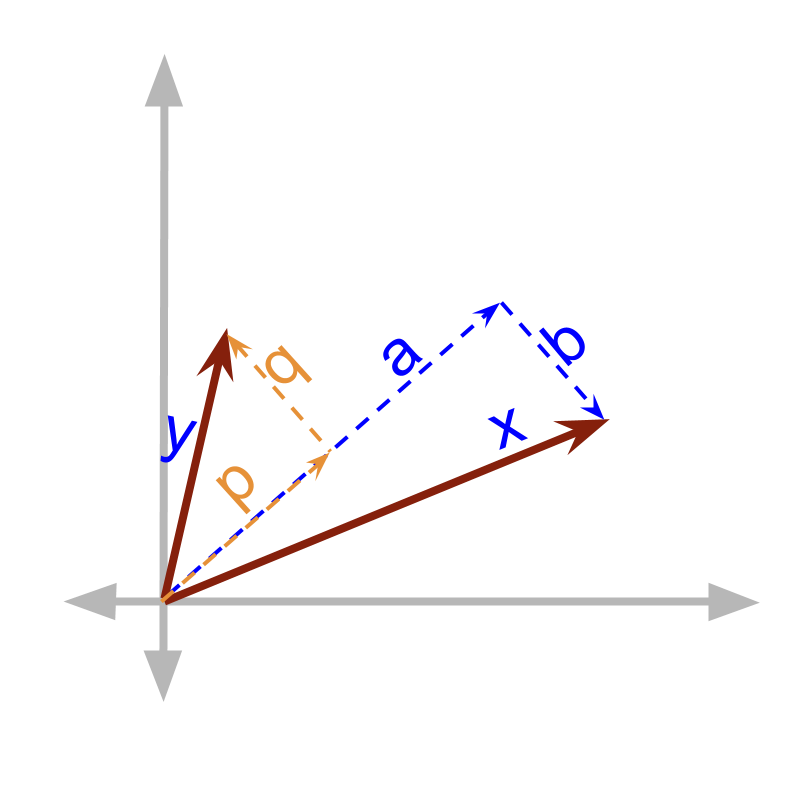The vectors $\stackrel{\to }{x}$$\vec{x}$ and $\stackrel{\to }{y}$$\vec{y}$ can be split into components
•  $\stackrel{\to }{x}$$\vec{x}$ = $\stackrel{\to }{a}$$\vec{a}$ + $\stackrel{\to }{b}$$\vec{b}$
•  $\stackrel{\to }{y}$$\vec{y}$ = $\stackrel{\to }{p}$$\vec{p}$ + $\stackrel{\to }{q}$$\vec{q}$

Note that $\stackrel{\to }{b}$$\vec{b}$ = $-\stackrel{\to }{q}$$- \vec{q}$
and $\stackrel{\to }{a}$$\vec{a}$ and $\stackrel{\to }{p}$$\vec{p}$ are in the same direction.The component vectors are equivalently rearranged as shown in the figure. $\stackrel{\to }{a}$$\vec{a}$ and $\stackrel{\to }{p}$$\vec{p}$ add in magnitude, as they are in the same direction.

The component vectors are equivalently rearranged as shown in the figure. $\stackrel{\to }{b}$$\vec{b}$ and $\stackrel{\to }{q}$$\vec{q}$ subtract in magnitude and cancel out to $0$$0$, as they are in the opposite direction and of same magnitude.

Note that the result forms a diagonal to the parallelogram. This is given as the parallelogram property of vector addition.

Two vectors form a parallelogram and the co-initial diagonal is the sum.

summary

Parallelogram Property of vector addition : when two vectors are added, arrange such that their initial points coincide. In that configuration, complete a parallelogram with the two vectors as the two adjacent sides. The diagonal that starts from the initial point of the vectors is the sum of the two vectors.

Outline